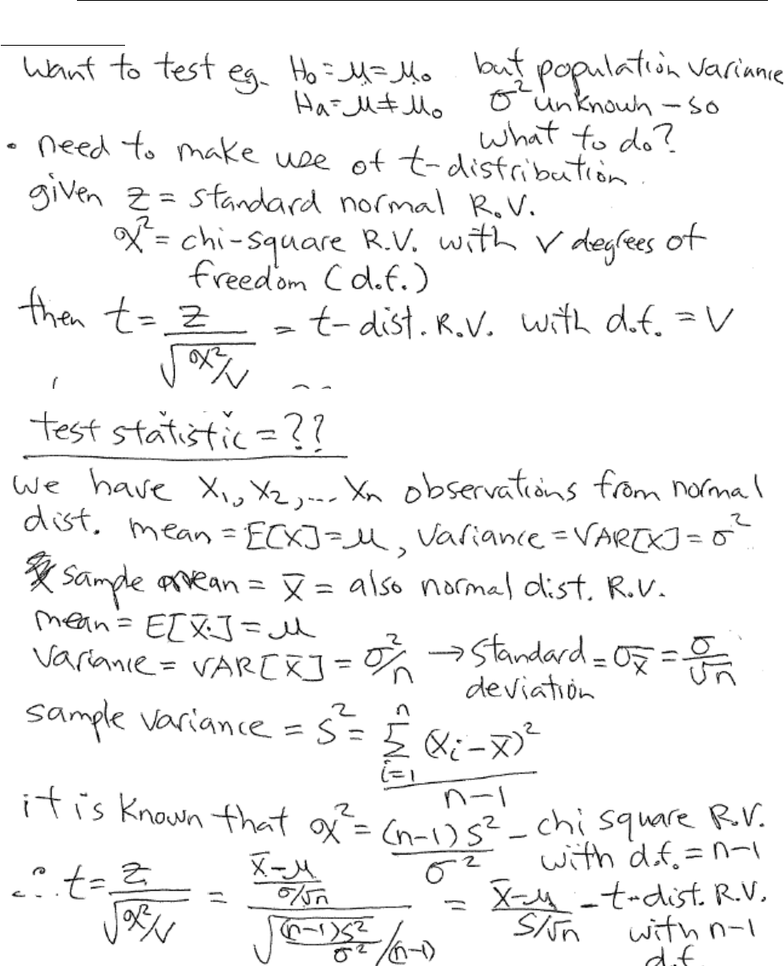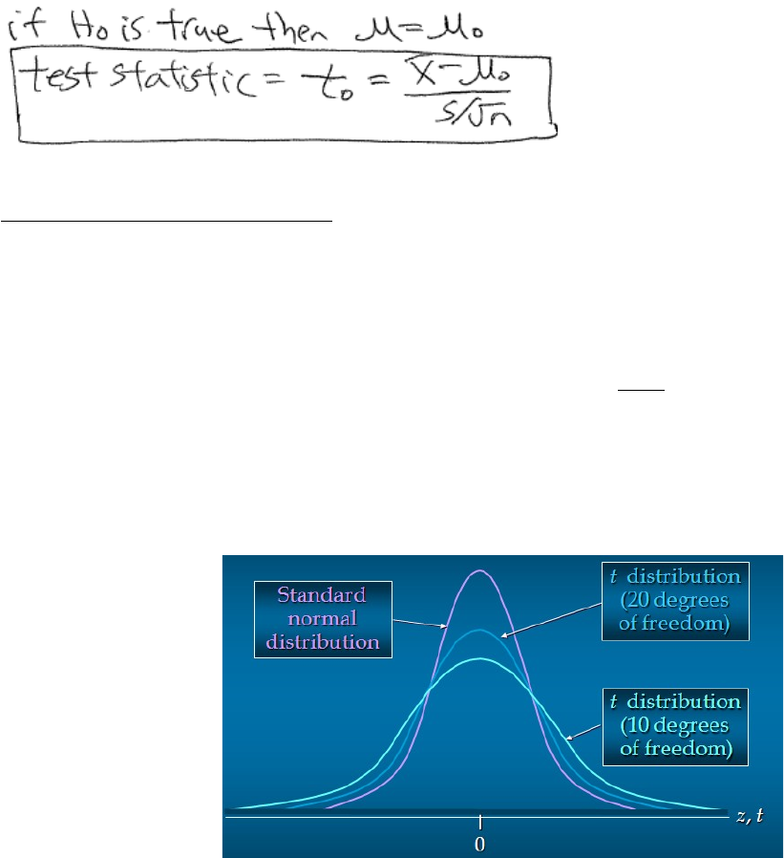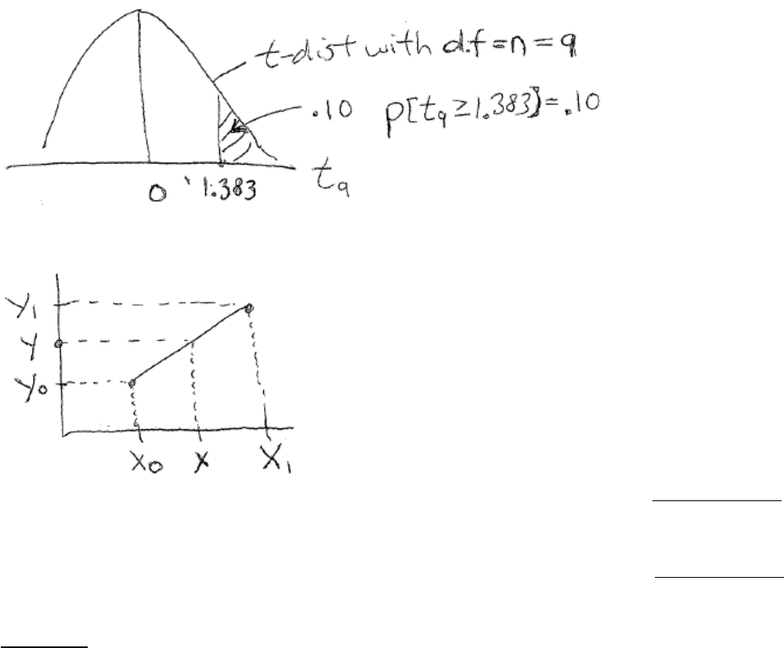Study Guides (390,000)
CA (150,000)
UTSC (10,000)
Midterm

# MGEB12H3 Study Guide - Midterm Guide: Stol, Random Variable, Joule

Department
Economics for Management Studies
Course Code
MGEB12H3
Professor
Victor Yu
Study Guide
Midterm

This preview shows pages 1-2. to view the full 8 pages of the document.Department of Management, UTSC
MGEB12 Quantitative Methods in Economics II - Lecture 02
Chapter 9 - Hypothesis Testing of Mean, Population Variance Unknown
1. Introduction
1

Only pages 1-2 are available for preview. Some parts have been intentionally blurred.2. t-Distributed Random Variable
t-distribution is symmetric just like normal distribution.
Degrees of freedom (d.f.) is # of independent pieces of information that goes into computing the standard
deviation s.
Expected value = E[t] = 0 Variance =
1
][
=
n
n
tVAR
where n = d.f.
The larger the degrees of freedom, the closer t-distribution resembles the standard normal distribution Z.
When d.f. n = 1000 it becomes practically standard normal Z.
Can use Table in Appendix B to lookup values of t-distribution.
oNormal distribution table Z gives lower (left) tail i.e. p[Z z]
ot-distribution table gives the upper (right) tail i.e., or p[tn-1 t]
2

Unlock to view full version

Only pages 1-2 are available for preview. Some parts have been intentionally blurred.Table in Appendix B only provides a limited number of values, may need to use Linear Interpolation to
Given 2 points (x0,y0) and (x1,y1) to find value of y for x0 ≤ x ≤ x1:
)(
))((
01
010
0
xx
yyxx
yy
+=
Given 2 points (x0,y0) and (x1,y1) to find value of x for y0 ≤ y ≤ y1:
)(
))((
01
010
0
yy
xxyy
xx
+=
Example 1
X is t-distributed with d.f. = 9.
P[ X ≥ 1.833] =
P[x ≤ 3.25] =
P[X ≥ 4] =
P[X ≥ .6] =
P[ X ≥ 2] =
3